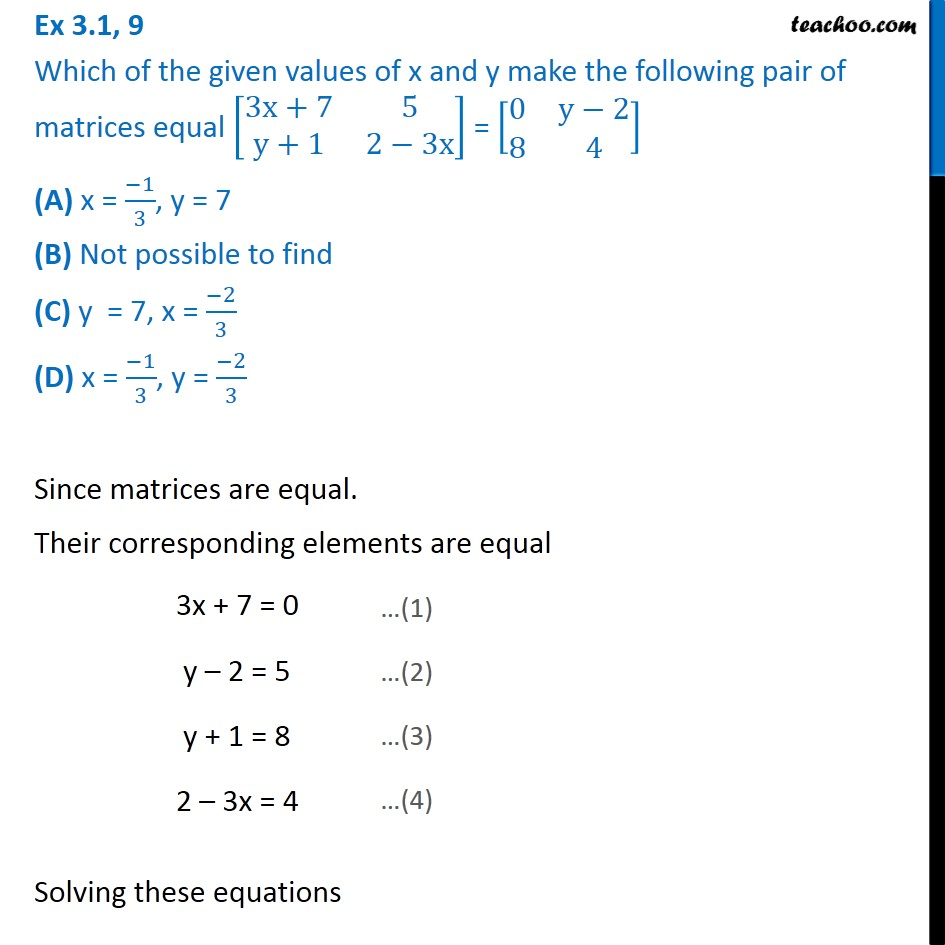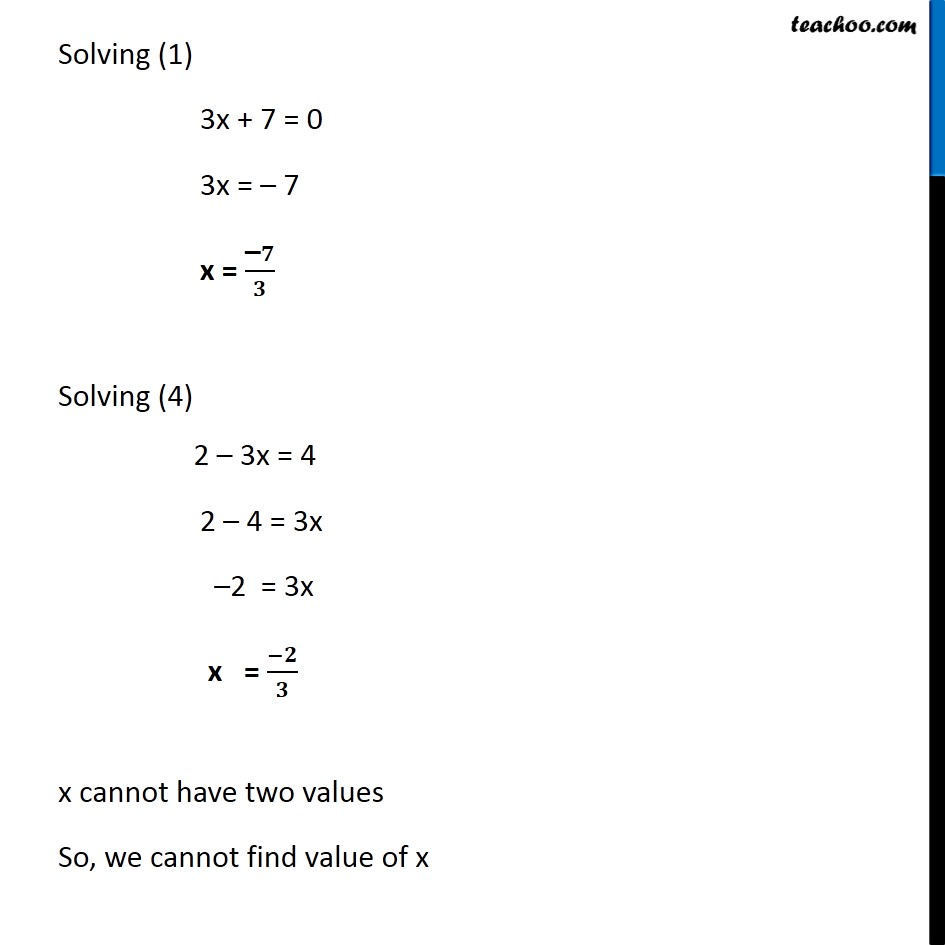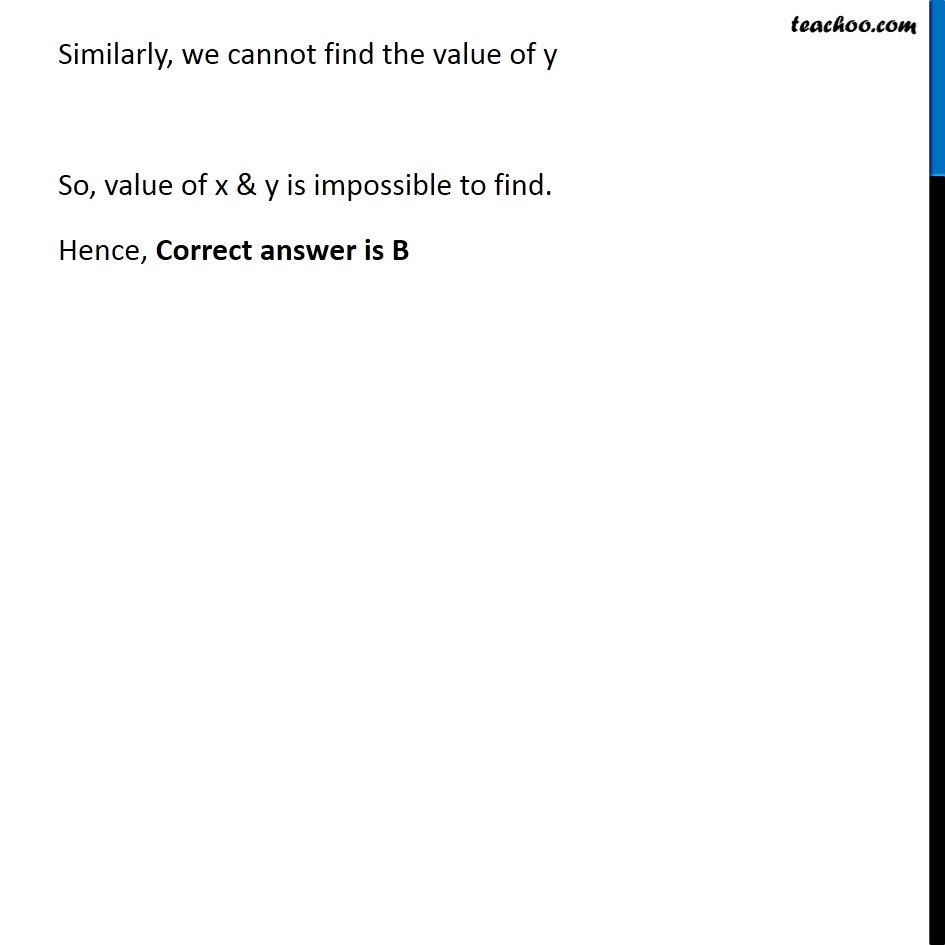1. Class 12
2. Important Questions for exams Class 12
3. Chapter 3 Class 12 Matrices

Transcript

Ex 3.1, 9 Which of the given values of x and y make the following pair of matrices equal [■8(3x+7&5@y+1&2−3x)] = [■8(0&y−2@8&4)] (A) x = (−1)/3, y = 7 (B) Not possible to find (C) y = 7, x = (−2)/3 (D) x = (−1)/3, y = (−2)/3 Since matrices are equal. Their corresponding elements are equal 3x + 7 = 0 y – 2 = 5 y + 1 = 8 2 – 3x = 4 Solving these equations …(1) …(1) …(3) …(4) Solving (1) 3x + 7 = 0 3x = – 7 x = ("–" 𝟕)/𝟑 Solving (4) 2 – 3x = 4 2 – 4 = 3x –2 = 3x x = (−𝟐)/𝟑 x cannot have two values So, we cannot find value of x Similarly, we cannot find the value of y So, value of x & y is impossible to find. Hence, Correct answer is B

Chapter 3 Class 12 Matrices

Class 12
Important Questions for exams Class 12